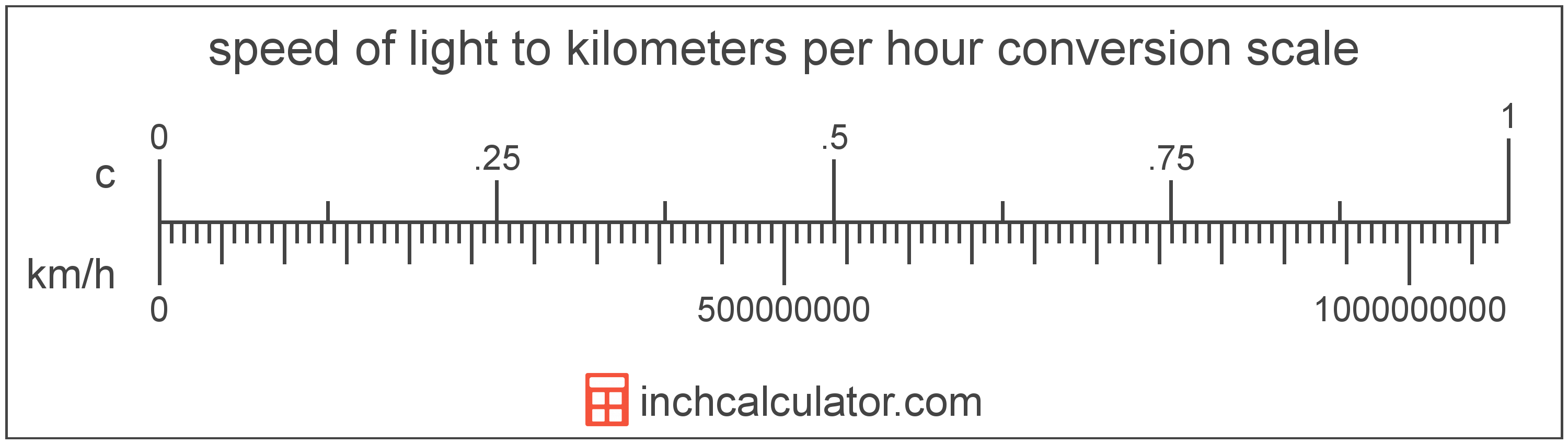# Kilometers Per Hour to Speed Of Light Conversion

Enter the speed in kilometers per hour below to get the value converted to speed of light.

Results in Speed Of Light:1 km/h = 9.2657E-10 c
Hint: use a scientific notation calculator to convert E notation to decimal

## How to Convert Kilometers Per Hour to Speed Of LightTo convert a kilometer per hour measurement to a speed of light measurement, multiply the speed by the conversion ratio.

Since one kilometer per hour is equal to 9.2657E-10 speed of light, you can use this simple formula to convert:

speed of light = kilometers per hour × 9.2657E-10

The speed in speed of light is equal to the kilometers per hour multiplied by 9.2657E-10.

For example, here's how to convert 5,000,000,000 kilometers per hour to speed of light using the formula above.
5,000,000,000 km/h = (5,000,000,000 × 9.2657E-10) = 4.632835 c

Kilometers per hour and speed of light are both units used to measure speed. Keep reading to learn more about each unit of measure.

## Kilometers Per Hour

Kilometers per hour are a measurement of speed expressing the distance traveled in kilometers in one hour.

The kilometer per hour, or kilometre per hour, is an SI unit of speed in the metric system. Kilometers per hour can be abbreviated as km/h, and are also sometimes abbreviated as kph. For example, 1 kilometer per hour can be written as 1 km/h or 1 kph.

In formal expressions, the slash, or solidus (/), is used to separate units used to indicate division in an expression.

Kilometers per hour can be expressed using the formula:
vkm/h = dkmthr

The velocity in kilometers per hour is equal to the distance in kilometers divided by time in hours.

## Speed Of Light

The speed of light is equal to exactly 299,792,458 meters per second, or 670,616,629 miles per hour. The definition of the speed of light is actually derived from the most recent 1983 international definition of the meter.

Speed of light can be abbreviated as c; for example, 1 speed of light can be written as 1 c.

## Kilometer Per Hour to Speed Of Light Conversion Table

Kilometer per hour measurements converted to speed of light
Kilometers Per Hour Speed Of Light
1 km/h 0.00000000092657 c
2 km/h 0.0000000018531 c
3 km/h 0.0000000027797 c
4 km/h 0.0000000037063 c
5 km/h 0.0000000046328 c
6 km/h 0.0000000055594 c
7 km/h 0.000000006486 c
8 km/h 0.0000000074125 c
9 km/h 0.0000000083391 c
10 km/h 0.0000000092657 c
100 km/h 0.000000092657 c
1,000 km/h 0.00000092657 c
10,000 km/h 0.0000092657 c
100,000 km/h 0.000092657 c
1,000,000 km/h 0.000927 c
10,000,000 km/h 0.009266 c
100,000,000 km/h 0.092657 c
1,000,000,000 km/h 0.926567 c
10,000,000,000 km/h 9.2657 c

## References

1. National Institute of Standards and Technology, NIST Guide to the SI, Chapter 6: Rules and Style Conventions for Printing and Using Units, https://www.nist.gov/pml/special-publication-811/nist-guide-si-chapter-6-rules-and-style-conventions-printing-and-using
2. National Institute of Standards and Technology, SI Redefinition: Meter, https://www.nist.gov/si-redefinition/meter